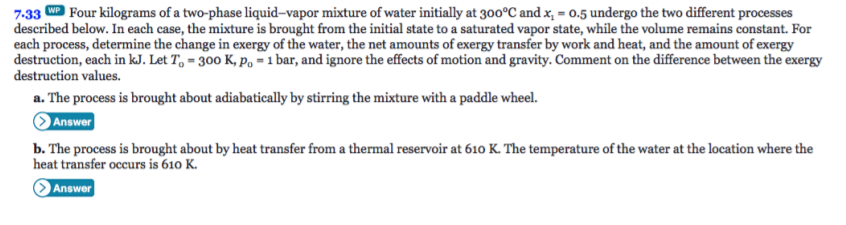# Four kilograms of a two-phase liquid-vapor mixture of water initially at 300°C and x, = 0.5 undergo the two different processes7.33described below. In each case, the mixture is brought from the initial state to a saturated vapor state, while the volume remains constant. Foreach process, determine the change in exergy of the water, the net amounts of exergy transfer by work and heat, and the amount of exergydestruction, each in kJ. Let To = 300 K, Po =1 bar, and ignore the effects of motion and gravity. Comment on the difference between the exergydestruction values.a. The process is brought about adiabatically by stirring the mixture with a paddle wheel.Answerb. The process is brought about by heat transfer from a thermal reservoir at 610 K. The temperature of the water at the location where theheat transfer occurs is 610 KAnswer

Question
29 viewshelp_outlineImage TranscriptioncloseFour kilograms of a two-phase liquid-vapor mixture of water initially at 300°C and x, = 0.5 undergo the two different processes 7.33 described below. In each case, the mixture is brought from the initial state to a saturated vapor state, while the volume remains constant. For each process, determine the change in exergy of the water, the net amounts of exergy transfer by work and heat, and the amount of exergy destruction, each in kJ. Let To = 300 K, Po =1 bar, and ignore the effects of motion and gravity. Comment on the difference between the exergy destruction values. a. The process is brought about adiabatically by stirring the mixture with a paddle wheel. Answer b. The process is brought about by heat transfer from a thermal reservoir at 610 K. The temperature of the water at the location where the heat transfer occurs is 610 K Answer fullscreen
check_circle

Step 1

Given:

T1 = 300°C

X1 = 0.5

X2 = 1.0

T0 = 300 K

P0 = 1 bar

Assumptions:

1. The water is a closed system.
2. The volume is constant.
3. In case (a); Q =0 and in case (b); W=0.
4. Kinetics and potential effects are negligible.

For both the cases the initial and final states are same. Let us begin by obtaining data at each state. From the thermodynamic table at temperature 300°C.

Step 2

From the assumptions (1) and (2); v2 = v1= vg(T2)

From the thermodynamic table

T2 = 336.8°C

U2 = 2474.2 KJ/Kg

S2 = 5.3673 KJ/Kg

Step 3

For part (a): In this case, the volume is constant. ...

### Want to see the full answer?

See Solution

#### Want to see this answer and more?

Solutions are written by subject experts who are available 24/7. Questions are typically answered within 1 hour.*

See Solution
*Response times may vary by subject and question.
Tagged in

### Heat Transfer